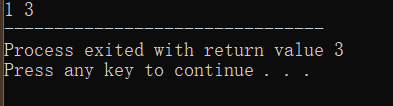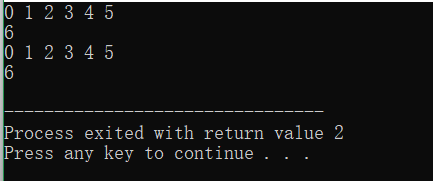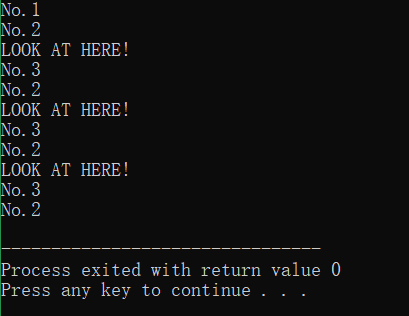# 在普通语句定义并用printf函数输出结果

void main()
{
int i = 1;
int a = i++;
//等价于 a = i; i = i + 1;
int b = ++i;
//等价于 i = i + 1; b = i;
printf("%d %d" ,a,b);
}# for循环中作为判断条件

void main()
{
int count = 0;
int count1 = 0;
for(int i = 0; i < 6; i++)
{
printf("%d ", i);
count++;
}
printf("\n");
printf("%d\n" , count);
for(int i = 0; i < 6; ++i)
{
printf("%d ", i);
count1++;
}
printf("\n");
printf("%d\n" , count1);
}void main()
{
int i;
for(printf("No.1\n"),i = 0; printf("No.2\n"),i < 3; printf("No.3\n"),i++)
{
printf("LOOK AT HERE! \n");
}
}# 结语

## Reference